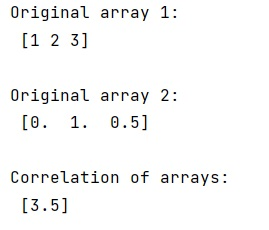# How can I use numpy.correlate() to do autocorrelation?

Learn, how to use numpy.correlate() to do autocorrelation in Python?
Submitted by Pranit Sharma, on January 02, 2023

NumPy is an abbreviated form of Numerical Python. It is used for different types of scientific operations in python. Numpy is a vast library in python which is used for almost every kind of scientific or mathematical operation. It is itself an array which is a collection of various methods and functions for processing the arrays.

## Using numpy.correlate() to do autocorrelation

Suppose need to do auto-correlation of a set of numbers, which is just the correlation of the set with itself.

There is always some kind of similarity/difference between all the values of all the different arrays. This similarity or difference is known as correlation of values in an array. To find the correlation in numpy, we use numpy.correlate() method.

This function computes the correlation as generally defined in signal processing texts:

ck=∑nan+k⋅v¯n

with a and v sequences being zero-padded where necessary and denoting complex conjugation.

Let us understand with the help of an example,

## Python code to demonstrate the use numpy.correlate() to do autocorrelation

```# Import numpy
import numpy as np

# Creating two numpy arrays
arr1 = np.array([1, 2, 3])
arr2 = np.array([0, 1, 0.5])

# Display original arrays
print("Original array 1:\n",arr1,"\n")
print("Original array 2:\n",arr2,"\n")

# Find the Correlation
res = np.correlate(arr1,arr2)

# Display result
print("Correlation of arrays:\n",res)
```

Output: